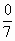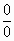Home MonkeyNotes Printable Notes Digital Library Study Guides Study Smart Parents Tips College Planning Test Prep Fun Zone Help / FAQ How to Cite New Title Request

1.3 Some properties of Basic Mathematical Operations

Closure : Suppose j is any ’Binary’ operation. A system is said to be closed for (or with respect to) j if a j b always fall into the system (original set) for any two elements a and b belonging to the system (original set).

(I) (1) The set (N) of natural numbers is closed under addition.

e.g. 1 + 3 = 4, 1, 3 and 4 Î N.

But it is not closed under subtraction.

e.g. 3 - 8 = - 5, 3, 8 Î N But - 5 Ï N.

(2) The set of even numbers is closed under addition.

e.g. 4 + 6 = 10, 4, 6 and 10 are even numbers.

The set of even numbers does not close for subtraction.

e.g. 6 - 8 = -2, 6, 8 are even numbers but -2 is not.

(3) The set of odd numbers is not closed for both addition and subtraction.

e.g. 3 + 5 = 8, 3, 5 are odd numbers but 8 is an even number.

(4) The set of rational numbers is closed under addition and subtraction.

e.g.etc.

(5) The set of real numbers is closed under addition and subtraction.(II) (1) The set of natural numbers is closed under multiplication.

e.g. 2 ´ 3 = 6

(2) The set of even numbers as well as the set of odd numbers are closed under multiplication.

e.g. (i) 4 ´ 6 = 24 (ii) 3 ´ 5 = 15

(3) The set of integers is closed under multiplication.

e.g. (i) 3 ´ 7 = 21 (ii) -3 ´ 5 = - 15 (iii) - 8 ´ -7 = 56

(4) The set of rational numbers is closed under multiplication.

(5) The set of real numbers is closed under multiplication.

Commutative : A binary operation * is said to be commutative on any system if for any 'a' and 'b' of the system, a * b = b * a.

1. Addition is commutative in the set of natural numbers, odd and even numbers,inintegers, in rational and in real numbers. i.e. a + b = b + a

2. Commutative does not hold for subtraction.

e.g. (i) 2 - 5 ą 5 - 2 (ii) a - b ą b - a

3. Multiplication is commutative in the set of natural numbers, integers, rational numbers and in real numbers.

(IV) Commutative does not hold for division.

e.g. 2 ¸ 4 ą 4 ¸ 2

Associative : A property showing that an operation is independent of the mode of grouping elements (Parentheses moved). Thus a binary operation * is said to be associative if for any three elements a, b, c of a system a * (b * c) = (a * b) * c

(I) The operations of addition and multiplication are associative over the set of numbers.

(i) (2 ´ 3) ´ 4 = 2 ´ (3 ´ 4)

(a ´ b) ´ c = a ´ (b ´ c)

(ii) (2 + 3) + 4 = 2 + (3 + 4)

(a + b) + c = a + (b + c)

(II) The operations of subtraction and division are not associative.

(i) 4 - (3 - 1) ą (4 - 3) - 1

a - (b - c) ą (a - b) - c

(ii) (8 ¸ 4) ¸ 2 ą 8 ¸ (4 ¸ 2)

(a ¸ b) ¸ c ą a ¸ (b ¸ c)

Existence of Identity Element

(I) Additive Identity : For addition the identity element is zero. Any number added to ‘O’ gives the original number.

7 + 0 = 0 + 7 = 7

a + 0 = 0 + a = a

Note that O is the additive identity for all sets of numbers.

(II) Multiplicative Identity : For multiplication the identity element is 1. Any number multiplied by 1 gives the original number.

7 ´ 1 = 1 ´ 7 = 7

a ´ 1 = 1 ´ a = a

Note : 1 is the multiplicative identify for all sets of numbers.

Existence of Inverses

(I) Additive Inverse : It is the opposite (negative) of the given number. Any number plus its additive inverse equals to 0 (the additive identity).

4 + (- 4) = - 4 + (4) = 0

\ 4 and - 4 are additive inverses of each other.

a + (- a) = - a + a = 0

In general a + b = b + a = 0 then a and b are additive inverses (or opposite numbers) of each other.

(II) Multiplicative Inverse : It is the reciprocal of the number. Any number multiplied by its reciprocal equals 1. In other words, corresponding to each number ‘a’, other than 0 there exists a number b such that ab = ba = 1

\The number b is the inverse (reciprocal ) of the number a written as b =or a-1

Since 1.1 = 1, therefore 1 is the inverse of itself i.e. 1-1 = 1

Distributive Property : A law which connects the operation of multiplication and addition. In general according to this property multiplication is distributive over the addition operation.

i.e. For any a, b, c , a ( b+ c) = ab + ac

OR (b + c) a = ba + ca

2 (3 +4) = 2 ´ 3 + 2 ´ 4

= 6 + 8 = 14

Note : Distributive property cannot be used with only one operation (See the definition)

4 (3 ´ 5 ´ 6) ą 4 ´ 3 ´ 4 ´ 5 ´ 4 ´ 6

The number pi (p) : It is a transcendental as well as an irrational number. The ratio of the circumference of any circle to its diameter is always constant and is denoted by pi (p). Its true value is 3.1415926536........ It was introduced by Archimedes who has shown that the exact value of p lies betweenand DTH=30 HEIGHT=41 align="middle">. For practicACE="Symbol">p =The number Zero :2. A place holder in arithmetical computation.

3. The ’Identity Element’ with respect to addition.

i.e. a + 0 = 0 + a = a

Multiplying and Dividing by zero

1. Zero times any number is equal to zero

\ 0 ´ 7 = 7 ´ 0 = 0

0 ´ (- 5) = (-5) ´ 0 = 02. Zero divided by any non-zero number is zero.

0 ¸ 5 = 0,= 0 , 0 ¸ (-2) = 0 etc.

3. Zero is the only number which cannot be a divisor i.e. division by zero is meaningless.

Note (1) division by zero is "undefined", thus not permitted at all.

(2)is one of the ’indeterminate’ forms.

Index

Introduction
1.1 Pre-requisties
1.2 Common Mathematical Symbols
1.3 Some Properties of Basic Mathematical Operations
1.4 Real Numbers

Chapter 2All Contents Copyright © All rights reserved.
Further Distribution Is Strictly Prohibited.

 Search: All Products Books Popular Music Classical Music Video DVD Toys & Games Electronics Software Tools & Hardware Outdoor Living Kitchen & Housewares Camera & Photo Cell Phones Keywords: